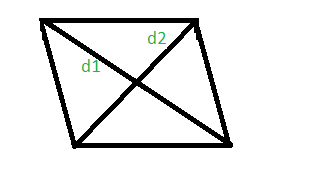GFG App
Open AppBrowser
Continue

# Program to calculate area and perimeter of a rhombus whose diagonals are given

Given the length of diagonals of a rhombus, d1 and d2. The task is to find the perimeter and the area of that rhombus.
A rhombus is a polygon having 4 equal sides in which both the opposite sides are parallel, and opposite angles are equal.Examples:

Input: d1 = 2 and d2 = 4
Output: The area of rhombus with diagonals 2 and 4 is 4.
The perimeter of rhombus with diagonals 2 and 4 is 8.

Input: d1 = 100 and d2 = 500
Output: The area of rhombus with diagonals 100 and 500 is 25000.
The perimeter of rhombus with diagonals 100 and 500 is 1019.

Area of the rhombus =Perimeterof the rhombus =Below is the implementation of above approach:

## C++

 // C++ Program to calculate area and perimeter // of a rhombus using diagonals #include  #include  using namespace std;   // calculate area and perimeter of a rhombus int rhombusAreaPeri(int d1, int d2) {     long long int area, perimeter;       area = (d1 * d2) / 2;     perimeter = 2 * sqrt(pow(d1, 2) + pow(d2, 2));       cout << "The area of rhombus with diagonals "          << d1 << " and " << d2 << " is " << area << "." << endl;       cout << "The perimeter of rhombus with diagonals "          << d1 << " and " << d2 << " is " << perimeter << "." << endl; }   // Driver code int main() {       int d1 = 2, d2 = 4;     rhombusAreaPeri(d1, d2);       return 0; }

## Java

 // Java program to calculate area and perimeter // of a rhombus using diagonals import java.io.*;   class GFG {     // calculate area and perimeter of a rhombus static int rhombusAreaPeri(int d1, int d2) {      int area, perimeter;       area = (d1 * d2) / 2;     perimeter = (int)(2 * Math.sqrt(Math.pow(d1, 2) + Math.pow(d2, 2)));       System.out.println( "The area of rhombus with diagonals "         + d1 + " and " + d2 + " is " + area + ".");       System.out.println("The perimeter of rhombus with diagonals "         +d1 + " and " + d2 + " is " + perimeter + ".");         return 0; }   // Driver code         public static void main (String[] args) {         int d1 = 2, d2 = 4;     rhombusAreaPeri(d1, d2);     } } // This code is contributed by anuj_67..

## Python3

 # Python 3 Program to calculate  # area and perimeter of a rhombus # using diagonals from math import sqrt, pow   # calculate area and perimeter # of a rhombus def rhombusAreaPeri(d1, d2):     area = (d1 * d2) / 2     perimeter = 2 * sqrt(pow(d1, 2) +                          pow(d2, 2))       print("The area of rhombus with diagonals",                  d1, "and", d2, "is", area, ".")       print("The perimeter of rhombus with diagonals",                 d1, "and", d2, "is", perimeter, "." )   # Driver code if __name__ == '__main__':     d1 = 2     d2 = 4     rhombusAreaPeri(d1, d2)   # This code is contributed  # by Surendra_Gangwar

## C#

 // C# program to calculate area  // and perimeter of a rhombus  // using diagonals using System;   class GFG  {   // calculate area and perimeter  // of a rhombus static int rhombusAreaPeri(int d1,                              int d2) {     int area, perimeter;       area = (d1 * d2) / 2;     perimeter = (int)(2 * Math.Sqrt(Math.Pow(d1, 2) +                                      Math.Pow(d2, 2)));       Console.WriteLine( "The area of rhombus with " +                         "diagonals " + d1 + " and " +                            d2 + " is " + area + ".");       Console.WriteLine("The perimeter of rhombus " +                        "with diagonals " + d1 + " and " +                          d2 + " is " + perimeter + ".");         return 0; }   // Driver code public static void Main ()  {     int d1 = 2, d2 = 4;     rhombusAreaPeri(d1, d2); } }   // This code is contributed by anuj_67..

## PHP

 

## Javascript

 

Output:

The area of rhombus with diagonals 2 and 4 is 4.
The perimeter of rhombus with diagonals 2 and 4 is 8.

Time Complexity: O(log(d12+d22))
Auxiliary Space: O(1), since no extra space has been taken.

My Personal Notes arrow_drop_up